Sunday, September 22, 2019
Home > Latest Announcement > Math Question With Solution Part 2: AMBQID 31 to 60

# Math Question With Solution Part 2: AMB QID 31

ABCD is a rectangle formed by 5 squares each of area 1 sq. units. BD is a diagonal. Find the shaded area (in sq. units).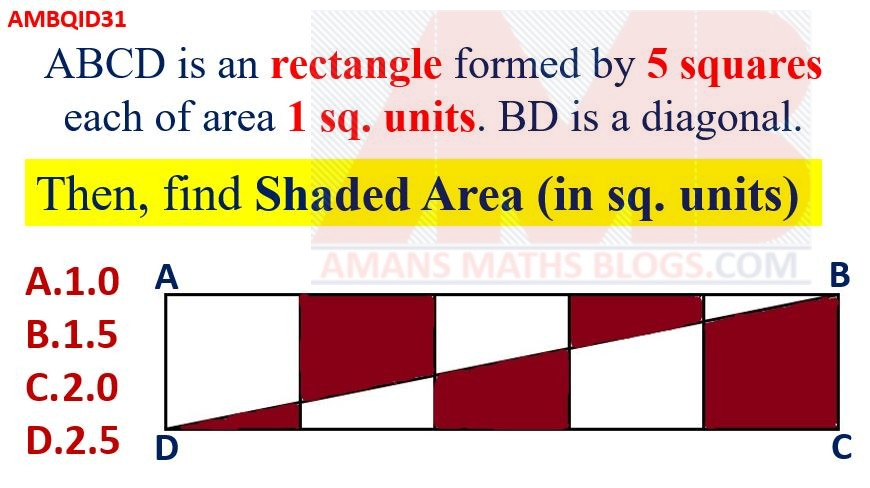Options:

A. 1.0

B. 1.5

C. 2.0

D. 2.5

Solution:

# Math Question With Solution Part 2: AMB QID 32

If x = 99, then find the value of x(x2 + 3x + 3).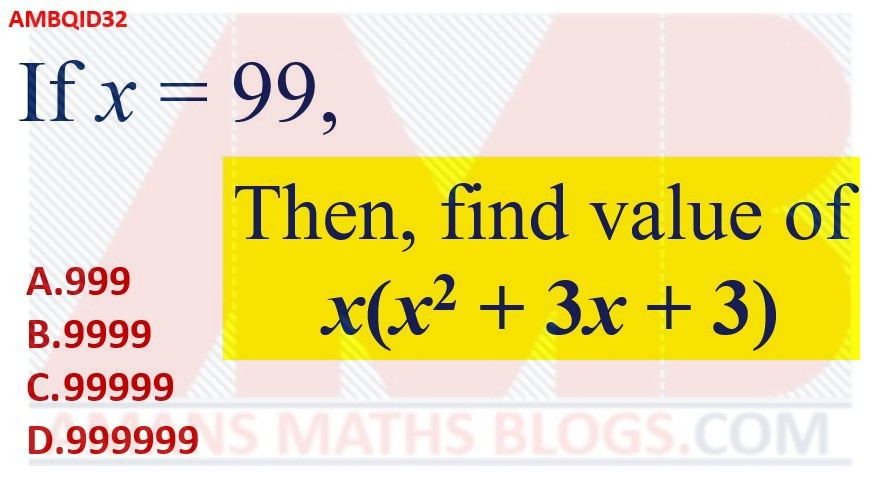Options:

A. 999

B. 9999

C. 99999

D. 999999

Solution:

# Math Question With Solution Part 2: AMB QID 33

If the central angle of a sector is 60 degree whose radius is OA = OB = 6 units, then find the area of circle inscribed in a sector.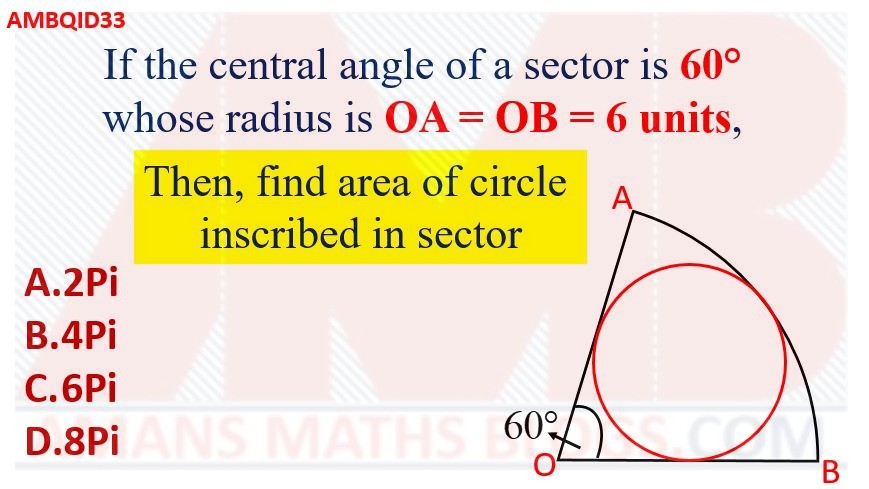Options:

A. 2Pi

B. 4Pi

C. 6Pi

D. 8Pi

Solution:

# Math Question With Solution Part 2: AMB QID 34

ABCD is a rectangle. If PC = 8 units, then find the area of rectangle ABCD.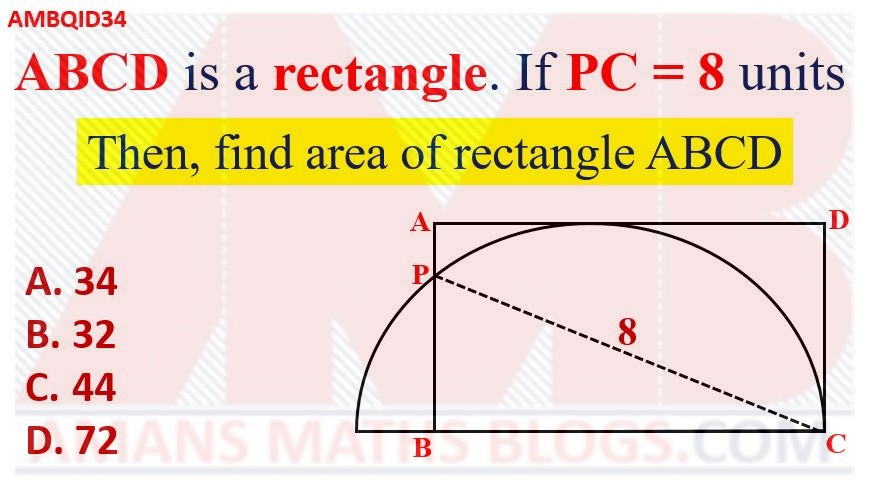Options:

A. 34

B. 32

C. 44

D. 72

Solution: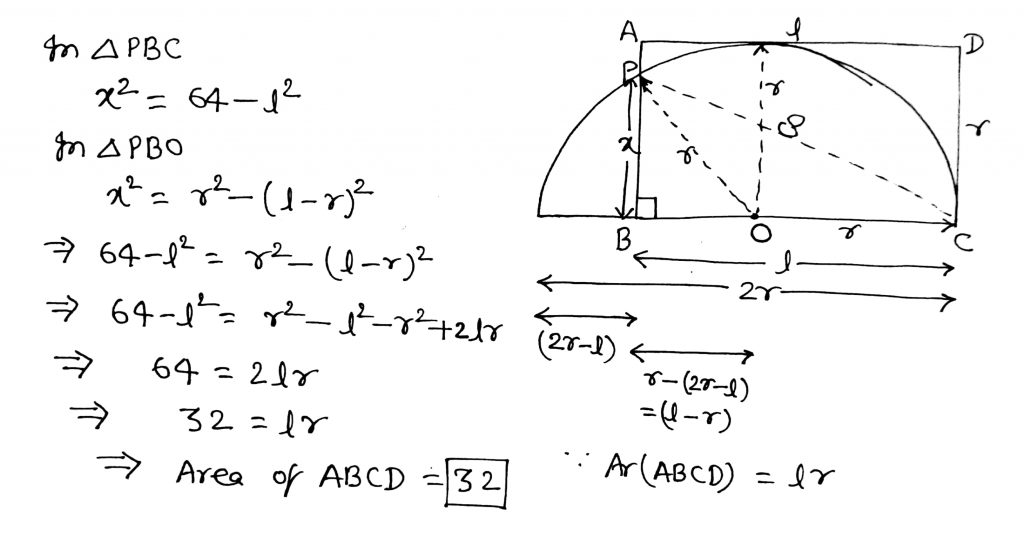error: Content is protected !!